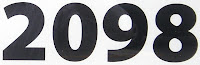## Wednesday, June 8, 2011

### 2098

2098 = 2 x 1049. It is a semiprime with a prime sum of factors: 2 + 1049 = 1051, a prime. It is also a semiprime one less than a prime: 2098 + 1 = 2099, a prime. The sum of its digits is a prime: 2 + 0 + 9 + 8 = 19, a prime.

2098 has a representation as a sum of two squares: 2098 = 272 + 372.

2098 is 747 in base 17.

2098 is the sum of 12 sixth powers.A transit of Mercury (its passage across the sun) is expected to occur in the year 2098.